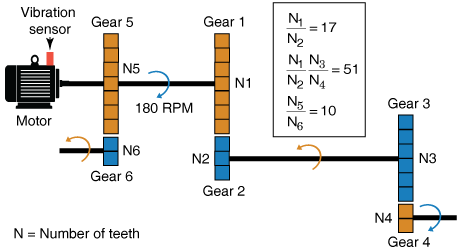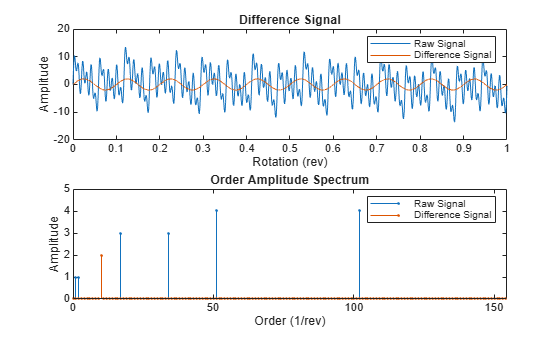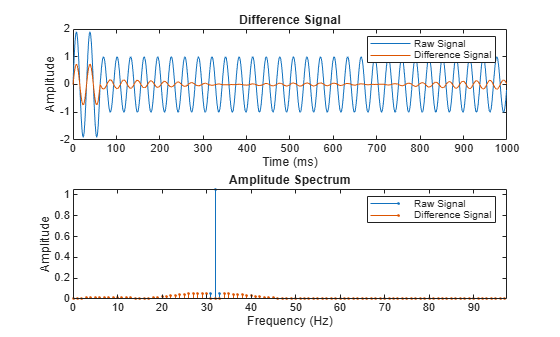Difference signal of a time-synchronous averaged signal

Since R2018b

## Syntax

``Y = tsadifference(X,fs,rpm,orderList)``
``Y = tsadifference(X,t,rpm,orderList)``
``Y = tsadifference(XT,rpm,orderList)``
``[Y,S] = tsadifference(___)``
``___ = tsadifference(___)``
``tsadifference(___)``

## Description

example

````Y = tsadifference(X,fs,rpm,orderList)` computes the difference signal `Y` of the time-synchronous averaged (TSA) signal vector `X` using sampling rate `fs`, the rotational speed `rpm`, and the orders to be filtered `orderList`. `Y` is computed by removing the regular signal, the value of '`NumSidebands`', and their respective harmonics from `X`. For more information on regular signal, see `tsaregular`.You can use `Y` to further extract condition indicators of rotating machinery for predictive maintenance. For example, extracting the FM4 indicator from `Y` is useful to detect faults isolated to only a limited number of teeth in a gear mesh.```

example

````Y = tsadifference(X,t,rpm,orderList)` computes the difference signal `Y` of the TSA signal vector `X` with corresponding time values from `t`.```

example

````Y = tsadifference(XT,rpm,orderList)` computes the difference signal `Y` of the TSA signal stored in the timetable `XT`. `XT` must contain a single numeric column variable.```

example

````[Y,S] = tsadifference(___)` returns the amplitude spectrum `S` of the difference signal `Y`. `S` is the amplitude spectrum computed using the normalized fast Fourier transform (FFT) of `Y`.```

example

````___ = tsadifference(___)` allows you to specify additional parameters using one or more name-value pair arguments. You can use this syntax with any of the previous input and output arguments.```

example

````tsadifference(___)` with no output arguments plots the time-domain and frequency-domain plots of the raw and difference TSA signals.```

## Examples

collapse all

Consider a drivetrain with six gears driven by a motor that is fitted with a vibration sensor, as depicted in the figure below. Gear 1 on the motor shaft meshes with gear 2 with a gear ratio of 17:1. The final gear ratio, that is, the ratio between gears 1 and 2 and gears 3 and 4, is 51:1. Gear 5, also on the motor shaft, meshes with gear 6 with a gear ratio of 10:1. The motor is spinning at 180 RPM, and the sampling rate of the vibration sensor is 50 KHz. To obtain the signal containing just the meshing components for gears 5 and 6, filter out the components of the shaft rotation, gears 1 and 2 and, 3 and 4 by specifying their gear ratios of 17 and 51 in `orderList`. The signal components corresponding to the shaft rotation (order = 1) is always implicitly included in the computation.```rpm = 180; fs = 50e3; t = (0:1/fs:(1/3)-1/fs)'; % sample times orderList = [17 51]; f = rpm/60*[1 orderList 10];```

In practice, you would use measured data such as vibration signals obtained from an accelerometer. For this example, generate TSA signal `X`, which is the simulated data from the vibration sensor mounted on the motor.

```X = sin(2*pi*f(1)*t) + sin(2*pi*2*f(1)*t) + ... % motor shaft rotation and harmonic 3*sin(2*pi*f(2)*t) + 3*sin(2*pi*2*f(2)*t) + ... % gear mesh vibration and harmonic for gears 1 and 2 4*sin(2*pi*f(3)*t) + 4*sin(2*pi*2*f(3)*t) + ... % gear mesh vibration and harmonic for gears 3 and 4 2*sin(2*pi*10*f(1)*t); % gear mesh vibration for gears 5 and 6```

Compute the difference signal of the TSA signal using the sample time, rpm, and the mesh orders to be filtered out.

`Y = tsadifference(X,t,rpm,orderList);`

The output `Y` is a vector containing the gear mesh signal and harmonics for gears 5 and 6.

Visualize the difference signal, the raw TSA signal, and their amplitude spectrum on a plot.

`tsadifference(X,fs,rpm,orderList)`From the amplitude spectrum plot, observe the following components:

• The filtered component at the 17th order and its harmonic at the 34th order

• The second filtered component at the 51st order and its harmonic at the 102nd order

• The residual mesh components for gears 5 and 6 at the 10th order

• The filtered shaft component at the 1st and 2nd orders

• The amplitudes on the spectrum plot match the amplitudes of individual signals

In this example, `sineWavePhaseMod.mat` contains the data of a phase modulated sine wave. `XT` is a timetable with the sine wave data and `rpm` used is 60 RPM. The sine wave has a frequency of 32 Hz. To filter out the unmodulated sine wave and the sidebands of the phase modulating signal, use 32 as the `orderList`.

Load the data and the required variables.

```load('sineWavePhaseMod.mat','XT','rpm','orders') head(XT,4)```
``` Time Data ______________ _______ 0 sec 0 0.00097656 sec 0.2011 0.0019531 sec 0.39399 0.0029297 sec 0.57078 ```

Note that the time values in `XT` are strictly increasing, equidistant, and finite.

Compute the difference signal and its amplitude spectrum. Set the value of `'Domain'` to `'frequency'` since the orders are in Hz.

`[Y,S] = tsadifference(XT,rpm,orders,'Domain','frequency')`
```Y=1024×1 timetable Time Data ______________ __________ 0 sec 2.2849e-15 0.00097656 sec 0.046525 0.0019531 sec 0.091185 0.0029297 sec 0.13219 0.0039062 sec 0.1679 0.0048828 sec 0.19688 0.0058594 sec 0.21799 0.0068359 sec 0.23039 0.0078125 sec 0.2336 0.0087891 sec 0.22751 0.0097656 sec 0.21239 0.010742 sec 0.18888 0.011719 sec 0.15793 0.012695 sec 0.12081 0.013672 sec 0.079041 0.014648 sec 0.034303 ⋮ ```
```S = 1024×1 complex -0.0000 + 0.0000i 0.0000 + 0.0000i 0.0000 + 0.0000i 0.0000 + 0.0000i 0.0000 + 0.0000i -0.0000 - 0.0000i -0.0000 + 0.0000i 0.0000 + 0.0000i -0.0000 - 0.0000i 0.0000 + 0.0000i ⋮ ```

The output `Y` is a timetable that contains the difference signal, while `S` is a vector that contains the amplitude spectrum of the difference signal `Y`.

In this example, `sineWaveRectangularPulse.mat` contains the data of a sine wave modulated by a rectangular pulse. `X` is a vector with the modulated sine wave data obtained at a shaft speed of 60 RPM. The unmodulated sine wave has a frequency of 32 Hz and amplitude of 1.0 units.

Load the data, and plot the difference signal of the modulated TSA signal `X`. To obtain the difference signal, filter out the unmodulated sine wave and the sidebands of the modulation signal by specifying the frequency of 32 Hz in `orderList`. Set the value of `'Domain'` to `'frequency'`.

```load('sineWaveRectangularPulse.mat','X','t','rpm','orderList') tsadifference(X,t,rpm,orderList,'Domain','frequency');```From the plot, observe the waveform and amplitude spectrum of the difference and raw signals, respectively. Observe that the difference signal contains everything except:

• Unmodulated sine wave at 32 Hz

• First-order sidebands of the unmodulated sine wave at 31 Hz and 33 Hz, respectively

## Input Arguments

collapse all

Time-synchronous averaged (TSA) signal, specified as a vector. The time-synchronous averaged signal is computed from a long and relatively periodic raw signal through synchronization, resampling, and averaging. For more information on TSA signals, see `tsa`.

Time-synchronous averaging is a convenient method of background noise reduction in a spectrum of complex signals. It is effective in concentrating useful information that can be extracted from a time-domain signal for predictive maintenance. The synchronization typically requires a tachometer pulse signal in addition to the raw sensor data. The TSA signal depicts measurements at equally spaced angular positions over a single revolution of a shaft of interest.

Time synchronous averaged (TSA) signal, specified as a timetable. `XT` must contain a single numeric column variable corresponding to the TSA signal. Time values in `XT` must be strictly increasing, equidistant, and finite.

Sampling frequency of the TSA signal in Hertz, specified as a positive scalar.

Sample times of the TSA signal, specified as a positive scalar or a vector of positive values.

If `t` is:

• A positive scalar, it contains the time interval or duration between samples. You must specify `t` as a `duration` variable.

• A vector of positive values, it contains sample times corresponding to elements in `X`. The time values must be strictly increasing, equidistant, and finite. You can specify `t` as a `double` or `duration` variable.

Rotational speed of the shaft, specified as a positive scalar. `tsadifference` uses a bandwidth equal to the shaft speed and the value of '`NumSidebands`' around the frequencies of interest to compute `Y` from the TSA signal. Specify `rpm` in revolutions per minute. The signal components corresponding to this frequency, that is, `order = 1` are always filtered out.

Orders to be filtered out of the TSA signal, specified as a vector of positive values. Select the orders and harmonics to be filtered out of the TSA signal by observing them on the amplitude spectrum plot. For instance, specify `orderList` as the known mesh orders in a gear train to filter out the known components and their harmonics. For more information, see Visualize the Difference Signal and Amplitude Spectrum of a TSA Signal. Specify the units of `orderList` by selecting the appropriate value for '`Domain`'.

### Name-Value Arguments

Specify optional pairs of arguments as `Name1=Value1,...,NameN=ValueN`, where `Name` is the argument name and `Value` is the corresponding value. Name-value arguments must appear after other arguments, but the order of the pairs does not matter.

Before R2021a, use commas to separate each name and value, and enclose `Name` in quotes.

Example: `...,'NumSidebands',2`

Number of shaft and gear meshing frequency harmonics to be filtered, specified as the comma-separated pair consisting of '`NumHarmonics`' and a positive integer. Modify '`NumHarmonics`' if your TSA signal contains more than two known harmonics of components to be filtered.

Number of sidebands to be filtered from the `orderList` frequencies and their harmonics, specified as the comma-separated pair consisting of '`NumSidebands`' and a nonnegative integer. The width of sidebands is determined using `2*(rpm/60)*(NumSidebands+0.5)`. Modify '`NumSidebands`' based on the number of sidebands to be filtered from `X` as observed in the amplitude spectrum plot.

Number of shaft rotations in the TSA signal, specified as the comma-separated pair consisting of '`NumRotations`' and a positive integer. Modify '`NumRotations`' if your input `X` or `XT` contains data for more than one rotation of the driver gear shaft. The function uses '`NumRotations`' to determine the number of rotations to be shown on the x-axis of the plot. The filtering results in `Y` are not affected by this value.

Units of the `orderList` values, specified as the comma-separated pair consisting of '`Domain`' and one of the following:

• `'frequency'`, if the orders in `orderList` are specified as frequencies in units of Hertz.

• `'order'`, if the orders in `orderList` are specified as number of rotations relative to the value of `rpm`. For example, if the rotational speed of the driven gear is defined as a factor of the driver gear rpm, specify '`Domain`' as `'order'`. Also, choose `'order'` if you are comparing data obtained from machines operating at different speeds.

## Output Arguments

collapse all

Difference signal of the TSA signal, returned as:

The difference signal is computed by removing the regular signal, the first-order sidebands, the value of '`NumSidebands`', and their respective harmonics from `X`. You can use `Y` to further extract condition indicators of rotating machinery for predictive maintenance. For example, extracting the FM4 indicator from `Y` is useful to detect faults isolated to only a limited number of teeth in a gear mesh. For more information on how `Y` is computed, see Algorithms.

For more information on regular signal, see `tsaregular`.

Amplitude spectrum of the difference signal, returned as a vector. `S` is the normalized fast Fourier transform of the signal `Y`. `S` is the same length as the input TSA signal `X`. For more information on how `S` is computed, see Algorithms.

## Algorithms

Difference Signal

The difference signal is computed from the TSA signal by filtering the following from the signal spectrum:

• Shaft frequency and its harmonics

• Gear meshing frequencies and their harmonics

• First-order sidebands at the gear meshing frequencies and their harmonics

• Optionally, the sidebands specified in '`NumSidebands`' at the gear meshing frequencies and their harmonics

`tsadifference` uses a bandwidth equal to three times the shaft speed and the value of '`NumSidebands`', around the frequencies of interest, to compute `Y` from the TSA signal.

Amplitude Spectrum

The amplitude spectrum of the difference signal is computed as follows,

Here, `Y` is the difference signal.

 McFadden, P.D. "Examination of a Technique for the Early Detection of Failure in Gears by Signal Processing of the Time Domain Average of the Meshing Vibration." Aero Propulsion Technical Memorandum 434. Melbourne, Australia: Aeronautical Research Laboratories, Apr. 1986.

 Večeř, P., Marcel Kreidl, and R. Šmíd. "Condition Indicators for Gearbox Monitoring Systems." Acta Polytechnica 45.6 (2005), pages 35-43.

 Zakrajsek, J. J., Townsend, D. P., and Decker, H. J. "An Analysis of Gear Fault Detection Methods as Applied to Pitting Fatigue Failure Data." Technical Memorandum 105950. NASA, Apr. 1993.

 Zakrajsek, James J. "An investigation of gear mesh failure prediction techniques." National Aeronautics and Space Administration Cleveland OH Lewis Research Center, 1989. No. NASA-E-5049.